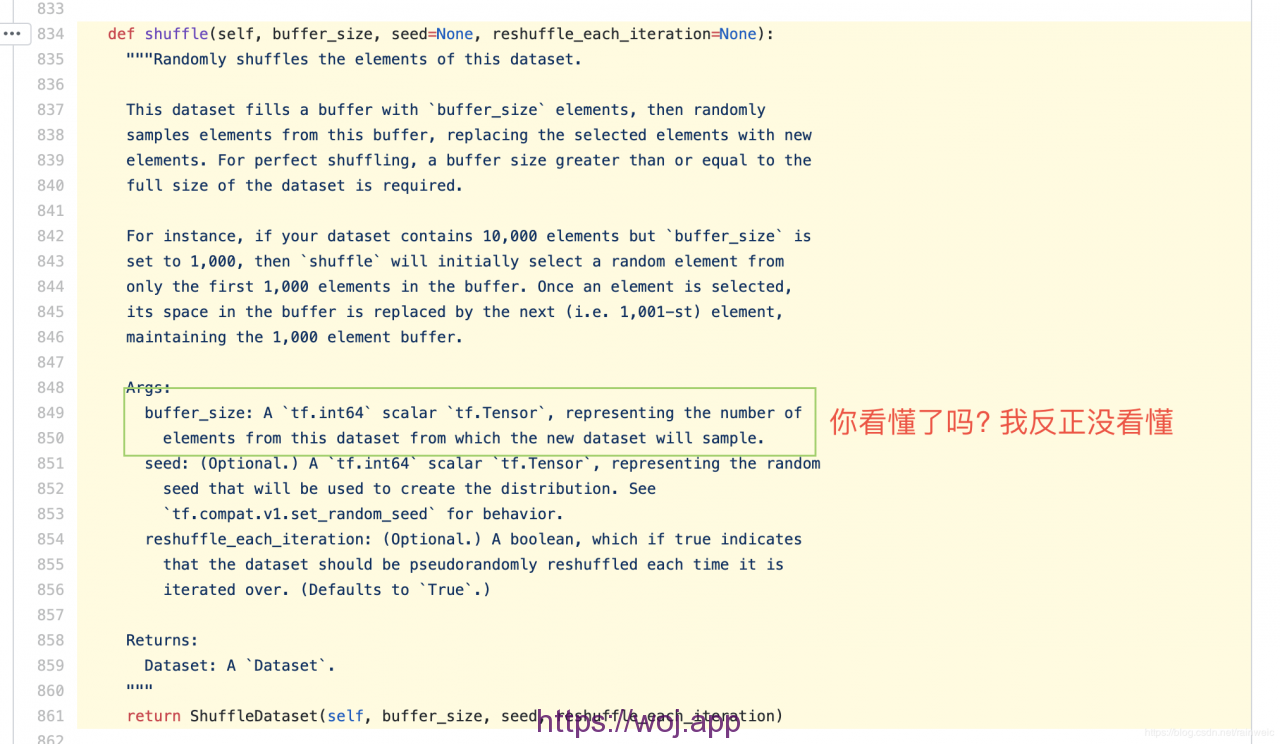A-A+

# 使用tf.data.Dataset.from_tensor_slices五步加载数据集

2020年04月30日 11:36 阅读 64 views 次

Step0: 准备要加载的numpy数据
Step1: 使用 tf.data.Dataset.from_tensor_slices() 函数进行加载
Step2: 使用 shuffle() 打乱数据
Step3: 使用 map() 函数进行预处理
Step4: 使用 batch() 函数设置 batch size 值
Step5: 根据需要 使用 repeat() 设置是否循环迭代数据集

```1 2 3 4 5 6 7 8 9 10 11 12 13 14 15 16 17 18 19 20 21 22 23 24 25 26 27 28 29 30 31 32 33 34 35 36 37 38 39 40 41 import tensorflow as tf from tensorflow import keras   def load_dataset(): # Step0 准备数据集, 可以是自己动手丰衣足食, 也可以从 tf.keras.datasets 加载需要的数据集(获取到的是numpy数据) # 这里以 mnist 为例 (x, y), (x_test, y_test) = keras.datasets.mnist.load_data()   # Step1 使用 tf.data.Dataset.from_tensor_slices 进行加载 db_train = tf.data.Dataset.from_tensor_slices((x, y)） db_test = tf.data.Dataset.from_tensor_slices((x_test, y_test))   # Step2 打乱数据 db_train.shuffle(1000) db_test.shuffle(1000)   # Step3 预处理 (预处理函数在下面) db_train.map(preprocess) db_test.map(preprocess)   # Step4 设置 batch size 一次喂入64个数据 db_train.batch(64) db_test.batch(64)   # Step5 设置迭代次数(迭代2次) test数据集不需要emmm db_train.repeat(2)   return db_train, db_test   def preprocess(labels, images): ''' 最简单的预处理函数: 转numpy为Tensor、分类问题需要处理label为one_hot编码、处理训练数据 ''' # 把numpy数据转为Tensor labels = tf.cast(labels, dtype=tf.int32) # labels 转为one_hot编码 labels = tf.one_hot(labels, depth=10) # 顺手归一化 images = tf.cast(images, dtype=tf.float32) / 255 return labels, images```

one_hot 编码: 小姐姐给你解释去 （我在使用自带的fit函数进行训练的时候，发现报错维度不正确，原来是不需要one_hot编码）

shuffle()函数的数值: 源码链接, 内容我贴图了### 布施恩德可便相知重

#### 微信扫一扫打赏#### 支付宝扫一扫打赏×